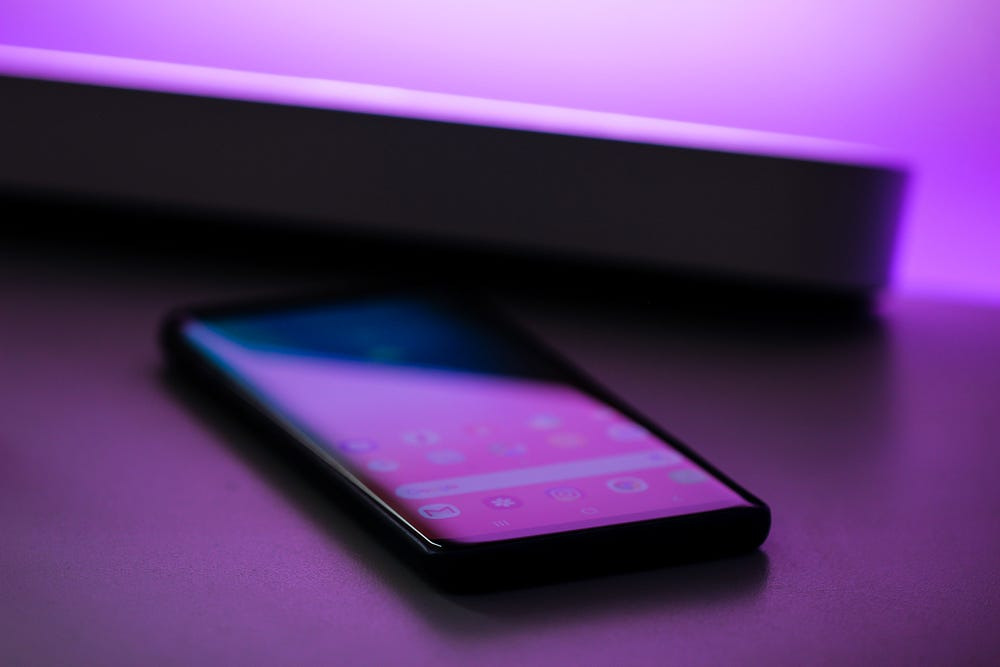# Sorting Dating Profiles with Machine Learning and Python

Original article can be found here (source): Artificial Intelligence on Medium# Sorting the Clustered Profiles

With the clustered profile data, we can further refine the results by sorting each profile based on how similar they are to one another. This process might be quicker and easier than you may think.

## Code Breakdown

Let’s break the code down to simple steps starting with `random`, which is used throughout the code simply to choose which cluster and user to select. This is done so that our code can be applicable to any user from the dataset. Once we have our randomly selected cluster, we can narrow down the entire dataset to just include those rows with the selected cluster.

## Vectorization

With our selected clustered group narrowed down, the next step involves vectorizing the bios in that group. The vectorizer we are using for this is the same one we used to create our initial clustered DataFrame — `CountVectorizer()`. (The vectorizer variable was instantiated previously when we vectorized the first dataset, which can be observed in the article above).

`# Fitting the vectorizer to the Bioscluster_x = vectorizer.fit_transform(group['Bios'])# Creating a new DF that contains the vectorized wordscluster_v = pd.DataFrame(cluster_x.toarray(),  index=group.index,  columns=vectorizer.get_feature_names())`

By vectorizing the Bios, we are creating a binary matrix that includes the words in each bio.

Afterwards, we will join this vectorized DataFrame to the selected group/cluster DataFrame.

`# Joining the vector DF and the original DFgroup = group.join(cluster_v)# Dropping the Bios because it is no longer neededgroup.drop('Bios', axis=1, inplace=True)`

After joining the two DataFrame together, we are left with vectorized bios and the categorical columns: